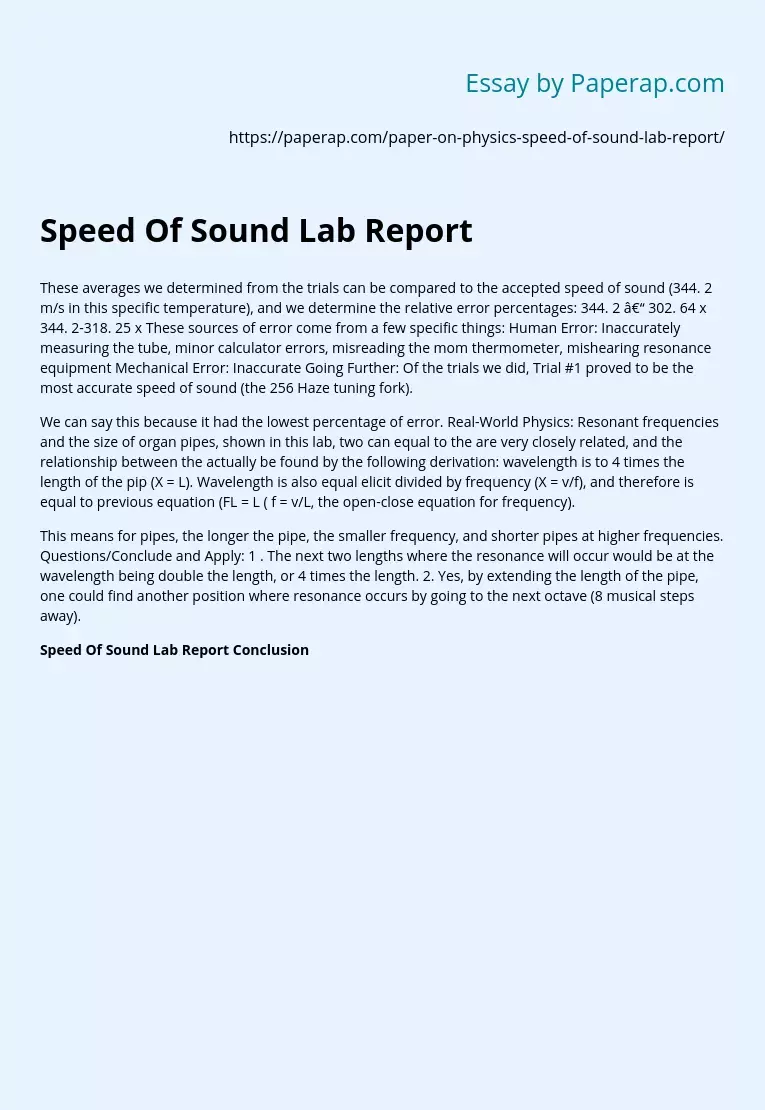# Speed Of Sound Lab Report

Topics: PhysicsSound

These averages we determined from the trials can be compared to the accepted speed of sound (344. 2 m/s in this specific temperature), and we determine the relative error percentages: 344. 2 – 302. 64 x 344. 2-318. 25 x These sources of error come from a few specific things: Human Error: Inaccurately measuring the tube, minor calculator errors, misreading the mom thermometer, mishearing resonance equipment Mechanical Error: Inaccurate Going Further: Of the trials we did, Trial #1 proved to be the most accurate speed of sound (the 256 Haze tuning fork).

We can say this because it had the lowest percentage of error. Real-World Physics: Resonant frequencies and the size of organ pipes, shown in this lab, two can equal to the are very closely related, and the relationship between the actually be found by the following derivation: wavelength is to 4 times the length of the pip (X = L). Wavelength is also equal elicit divided by frequency (X = v/f), and therefore is equal to previous equation (FL = L ( f = v/L, the open-close equation for frequency).

This means for pipes, the longer the pipe, the smaller frequency, and shorter pipes at higher frequencies. Questions/Conclude and Apply: 1 . The next two lengths where the resonance will occur would be at the wavelength being double the length, or 4 times the length. 2. Yes, by extending the length of the pipe, one could find another position where resonance occurs by going to the next octave (8 musical steps away).

## Speed Of Sound Lab Report Conclusion